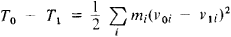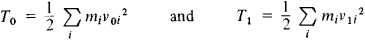# Carnot's Theorem

(redirected from Carnot theorem)

## Carnot's theorem

[kär′noz ′thir·əm]
(thermodynamics)
The theorem that all Carnot engines operating between two given temperatures have the same efficiency, and no cyclic heat engine operating between two given temperatures is more efficient than a Carnot engine.
The theorem that any system has two properties, the thermodynamic temperature T and the entropy S, such that the amount of heat exchanged in an infinitesimal reversible process is given by dQ = TdS; the thermodynamic temperature is a strictly increasing function of the empirical temperature measured on an arbitrary scale.
McGraw-Hill Dictionary of Scientific & Technical Terms, 6E, Copyright © 2003 by The McGraw-Hill Companies, Inc.
The following article is from The Great Soviet Encyclopedia (1979). It might be outdated or ideologically biased.

## Carnot’s Theorem

(1) The theorem of the efficiency of heat engines that was established by N. L. S. Carnot in 1824. According to the theorem, the efficiency of the Carnot cycle does not depend on the nature of the working substance or the design of the heat engine but is determined only by the temperatures of the heater and cooler. Carnot’s theorem played an important role in the establishment of the second law of thermodynamics.

(2) In impact theory, the theorem of the loss of kinetic energy during an absolutely inelastic impact. It is named for L. N. Carnot. The kinetic energy lost by a system upon impact is equal to the kinetic energy that the system would have had if its points had moved at the velocities lost, that iswhereare the values of the kinetic energy of the system before and afterimpact, respectively; mi is the mass of the i-th point; voi, and v1i, are the velocities of the i-th point before and after impact; and (v0i, — v1i) is the lost velocity of the point. Carnot’s theoremis a direct consequence of the application of the laws of conserva-tion of momentum and energy for an isolated mechanical systemto the phenomenon of inelastic impact. In many cases Carnot’stheorem makes possible the determination of the velocities of bodies after inelastic impact.